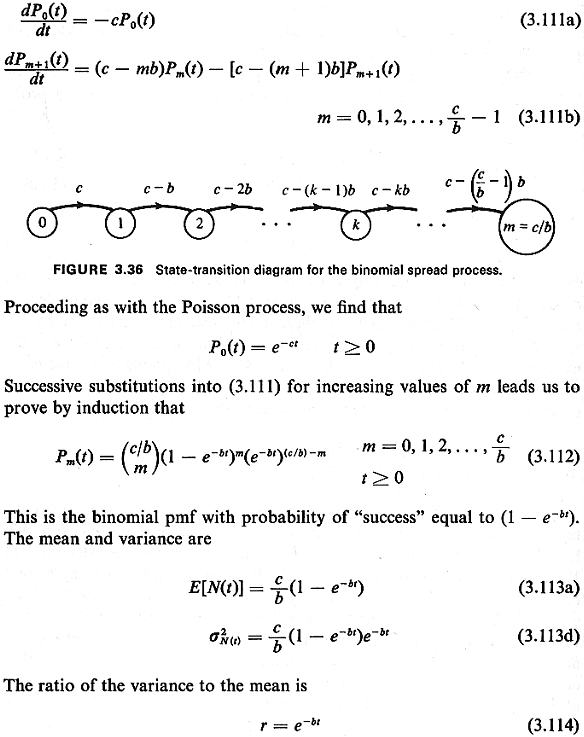## 3.9.1 Spread Process Yielding the Binomial PMF

Rogers has studied two particular processes--one spread and one clustered--that have appealing time-Poisson process interpretations and that have been found useful in analyzing the locations of retail trade [ROGE 74]. We consider first Rogers's spread process, the binomial process. Imagine that entities enter the cell of interest over some time interval [0, t], initially with 0 entities in the cell. We are interested in the number of entities at time t, N(t). Being a spread process, each time that another entity enters the cell the rate at which new entities enter the cell diminishes. Thus, suppose initially that entities enter the cell as a time-Poisson process at rate c per unit time. Then, after the first enters the cell, the cell becomes "less attractive," so the new Poisson arrival rate is c - b. In general, after k arrivals, the Poisson arrival rate is reduced to c - kb. Thus, the cell becomes less attractive in a linear manner with the number of entities already in the cell. We assume that c/b is integer, so that there exists some maximum k, kmax = c/b, at which the Poisson arrival rate is reduced to c - kmaxb = 0 Thus, the maximum number of entities in a cell is kmax = c/b. This pure birth process is characterized by the state-transition diagram shown in Figure 3.36.
Proceeding as in Chapter 2 for the Poisson process, this process is governed by the following set of coupled differential equations:

``````

which is always less than unity (which is what we want with a spread process).
While the "diminishing attractiveness" interpretation of this birth process is perfectly valid, and quite appealing as a description of the dynamics of a spread process, it is not the only interpretation of the process. Alternatively, one might imagine a population fixed with n = c/b individuals. Each one will eventually locate within the cell, but the time until such location is an exponentially distributed random variable with mean 1/b. All n such random variables are mutually independent. Thus, at time t = 0, n "Poisson generators" are turned on, yielding a rate of transition nb from state 0 to 1; after the first transition, (n - 1) Poisson generators remain turned on with a net rate of occurrence equal to (n - 1)b. This "fixed population" interpretation of cell occupancy also yields the binomial distribution, and it could imply markedly different policy decisions in practice than the "diminishing attractiveness" interpretation. The two equally plausible interpretations provide a good example that any particular probability law may have two, three, or even a greater number of plausible underlying explanations. Thus, just because a probability law assumes a particular form does not assure us that one underlying causal model is the model explaining the process dynamics.